This book is archived and will be removed July 6, 2022. Please use the updated version.

Electromagnetic Waves

# 110 Momentum and Radiation Pressure

### Learning Objectives

By the end of this section, you will be able to:

• Describe the relationship of the radiation pressure and the energy density of an electromagnetic wave
• Explain how the radiation pressure of light, while small, can produce observable astronomical effects

Material objects consist of charged particles. An electromagnetic wave incident on the object exerts forces on the charged particles, in accordance with the Lorentz force, (Figure). These forces do work on the particles of the object, increasing its energy, as discussed in the previous section. The energy that sunlight carries is a familiar part of every warm sunny day. A much less familiar feature of electromagnetic radiation is the extremely weak pressure that electromagnetic radiation produces by exerting a force in the direction of the wave. This force occurs because electromagnetic waves contain and transport momentum.

To understand the direction of the force for a very specific case, consider a plane electromagnetic wave incident on a metal in which electron motion, as part of a current, is damped by the resistance of the metal, so that the average electron motion is in phase with the force causing it. This is comparable to an object moving against friction and stopping as soon as the force pushing it stops ((Figure)). When the electric field is in the direction of the positive y-axis, electrons move in the negative y-direction, with the magnetic field in the direction of the positive z-axis. By applying the right-hand rule, and accounting for the negative charge of the electron, we can see that the force on the electron from the magnetic field is in the direction of the positive x-axis, which is the direction of wave propagation. When the E field reverses, the B field does too, and the force is again in the same direction. Maxwell’s equations together with the Lorentz force equation imply the existence of radiation pressure much more generally than this specific example, however.

Electric and magnetic fields of an electromagnetic wave can combine to produce a force in the direction of propagation, as illustrated for the special case of electrons whose motion is highly damped by the resistance of a metal.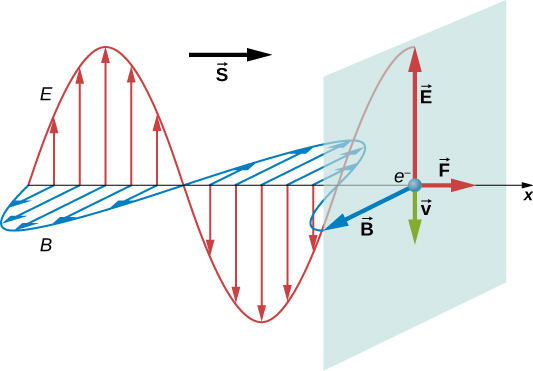Maxwell predicted that an electromagnetic wave carries momentum. An object absorbing an electromagnetic wave would experience a force in the direction of propagation of the wave. The force corresponds to radiation pressure exerted on the object by the wave. The force would be twice as great if the radiation were reflected rather than absorbed.

Maxwell’s prediction was confirmed in 1903 by Nichols and Hull by precisely measuring radiation pressures with a torsion balance. The schematic arrangement is shown in (Figure). The mirrors suspended from a fiber were housed inside a glass container. Nichols and Hull were able to obtain a small measurable deflection of the mirrors from shining light on one of them. From the measured deflection, they could calculate the unbalanced force on the mirror, and obtained agreement with the predicted value of the force.

Simplified diagram of the central part of the apparatus Nichols and Hull used to precisely measure radiation pressure and confirm Maxwell’s prediction.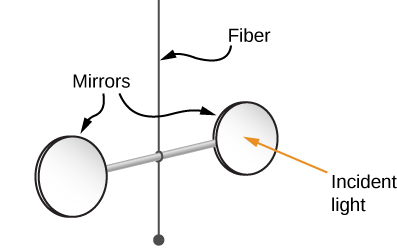The radiation pressure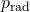applied by an electromagnetic wave on a perfectly absorbing surface turns out to be equal to the energy density of the wave: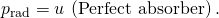If the material is perfectly reflecting, such as a metal surface, and if the incidence is along the normal to the surface, then the pressure exerted is twice as much because the momentum direction reverses upon reflection: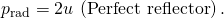We can confirm that the units are right: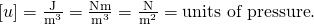(Figure) and (Figure) give the instantaneous pressure, but because the energy density oscillates rapidly, we are usually interested in the time-averaged radiation pressure, which can be written in terms of intensity: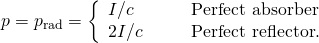Radiation pressure plays a role in explaining many observed astronomical phenomena, including the appearance of comets. Comets are basically chunks of icy material in which frozen gases and particles of rock and dust are embedded. When a comet approaches the Sun, it warms up and its surface begins to evaporate. The coma of the comet is the hazy area around it from the gases and dust. Some of the gases and dust form tails when they leave the comet. Notice in (Figure) that a comet has two tails. The ion tail (or gas tail in (Figure)) is composed mainly of ionized gases. These ions interact electromagnetically with the solar wind, which is a continuous stream of charged particles emitted by the Sun. The force of the solar wind on the ionized gases is strong enough that the ion tail almost always points directly away from the Sun. The second tail is composed of dust particles. Because the dust tail is electrically neutral, it does not interact with the solar wind. However, this tail is affected by the radiation pressure produced by the light from the Sun. Although quite small, this pressure is strong enough to cause the dust tail to be displaced from the path of the comet.

Evaporation of material being warmed by the Sun forms two tails, as shown in this photo of Comet Ison. (credit: modification of work by E. Slawik—ESO)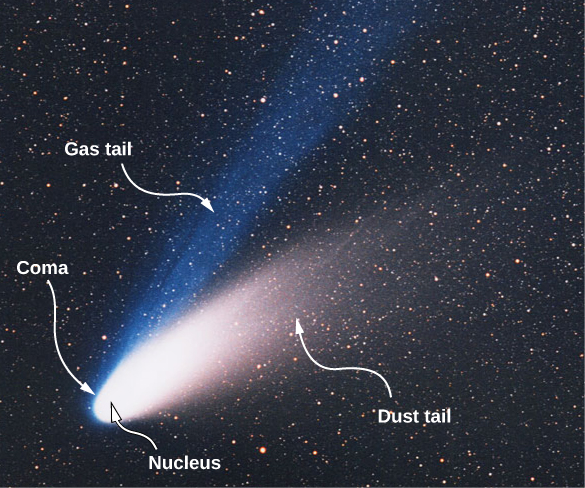Halley’s Comet On February 9, 1986, Comet Halley was at its closest point to the Sun, about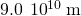from the center of the Sun. The average power output of the Sun is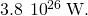(a) Calculate the radiation pressure on the comet at this point in its orbit. Assume that the comet reflects all the incident light.

(b) Suppose that a 10-kg chunk of material of cross-sectional area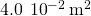breaks loose from the comet. Calculate the force on this chunk due to the solar radiation. Compare this force with the gravitational force of the Sun.

Strategy Calculate the intensity of solar radiation at the given distance from the Sun and use that to calculate the radiation pressure. From the pressure and area, calculate the force.

Solution

1. The intensity of the solar radiation is the average solar power per unit area. Hence, atfrom the center of the Sun, we have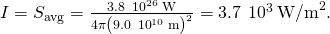Assuming the comet reflects all the incident radiation, we obtain from (Figure)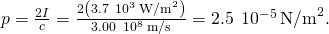2. The force on the chunk due to the radiation is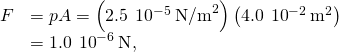whereas the gravitational force of the Sun is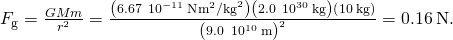Significance The gravitational force of the Sun on the chunk is therefore much greater than the force of the radiation.

After Maxwell showed that light carried momentum as well as energy, a novel idea eventually emerged, initially only as science fiction. Perhaps a spacecraft with a large reflecting light sail could use radiation pressure for propulsion. Such a vehicle would not have to carry fuel. It would experience a constant but small force from solar radiation, instead of the short bursts from rocket propulsion. It would accelerate slowly, but by being accelerated continuously, it would eventually reach great speeds. A spacecraft with small total mass and a sail with a large area would be necessary to obtain a usable acceleration.

When the space program began in the 1960s, the idea started to receive serious attention from NASA. The most recent development in light propelled spacecraft has come from a citizen-funded group, the Planetary Society. It is currently testing the use of light sails to propel a small vehicle built from CubeSats, tiny satellites that NASA places in orbit for various research projects during space launches intended mainly for other purposes.

The LightSail spacecraft shown below ((Figure)) consists of three CubeSats bundled together. It has a total mass of only about 5 kg and is about the size as a loaf of bread. Its sails are made of very thin Mylar and open after launch to have a surface area of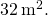Two small CubeSat satellites deployed from the International Space Station in May, 2016. The solar sails open out when the CubeSats are far enough away from the Station. (credit: modification of work by NASA)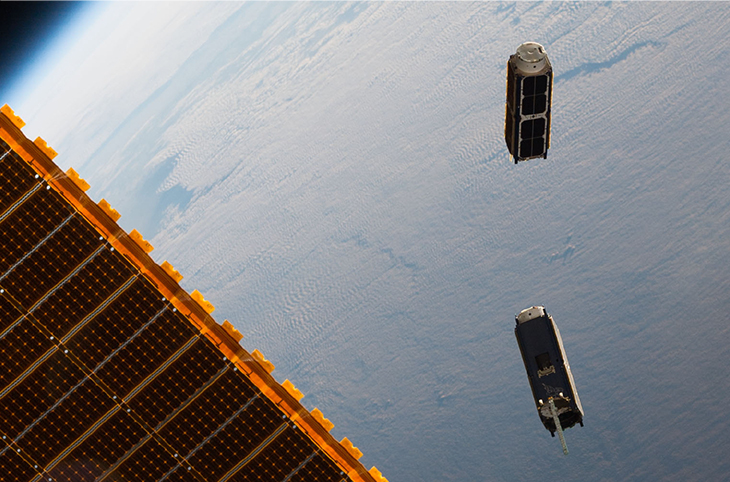The first LightSail spacecraft was launched in 2015 to test the sail deployment system. It was placed in low-earth orbit in 2015 by hitching a ride on an Atlas 5 rocket launched for an unrelated mission. The test was successful, but the low-earth orbit allowed too much drag on the spacecraft to accelerate it by sunlight. Eventually, it burned in the atmosphere, as expected. The next Planetary Society’s LightSail solar sailing spacecraft is scheduled for 2016. An illustration of the spacecraft, as it is expected to appear in flight, can be seen on the Planetary Society’s website.

LightSail Acceleration The intensity of energy from sunlight at a distance of 1 AU from the Sun is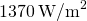. The LightSail spacecraft has sails with total area of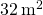and a total mass of 5.0 kg. Calculate the maximum acceleration LightSail spacecraft could achieve from radiation pressure when it is about 1 AU from the Sun.

Strategy The maximum acceleration can be expected when the sail is opened directly facing the Sun. Use the light intensity to calculate the radiation pressure and from it, the force on the sails. Then use Newton’s second law to calculate the acceleration.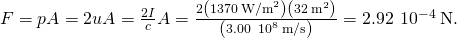The resulting acceleration is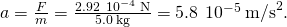Significance If this small acceleration continued for a year, the craft would attain a speed of 1829 m/s, or 6600 km/h.

Check Your Understanding How would the speed and acceleration of a radiation-propelled spacecraft be affected as it moved farther from the Sun on an interplanetary space flight?

Its acceleration would decrease because the radiation force is proportional to the intensity of light from the Sun, which decreases with distance. Its speed, however, would not change except for the effects of gravity from the Sun and planets.

### Summary

• Electromagnetic waves carry momentum and exert radiation pressure.
• The radiation pressure of an electromagnetic wave is directly proportional to its energy density.
• The pressure is equal to twice the electromagnetic energy intensity if the wave is reflected and equal to the incident energy intensity if the wave is absorbed.

### Conceptual Questions

Why is the radiation pressure of an electromagnetic wave on a perfectly reflecting surface twice as large as the pressure on a perfectly absorbing surface?

The force on a surface acting over timeis the momentum that the force would impart to the object. The momentum change of the light is doubled if the light is reflected back compared with when it is absorbed, so the force acting on the object is twice as great.

Why did the early Hubble Telescope photos of Comet Ison approaching Earth show it to have merely a fuzzy coma around it, and not the pronounced double tail that developed later (see below)?

(credit: modification of work by NASA, ESA, J.-Y. Li (Planetary Science Institute), and the Hubble Comet ISON Imaging Science Team)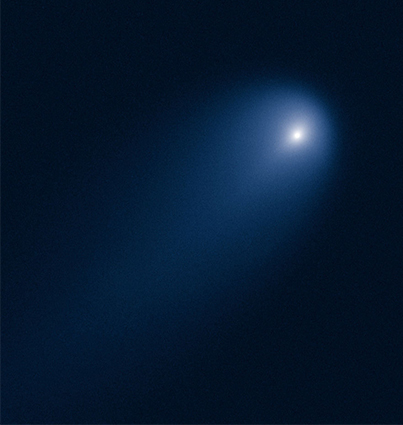(a) If the electric field and magnetic field in a sinusoidal plane wave were interchanged, in which direction relative to before would the energy propagate?
(b) What if the electric and the magnetic fields were both changed to their negatives?

a. According to the right hand rule, the direction of energy propagation would reverse. b. This would leave the vector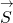, and therefore the propagation direction, the same.

### Problems

A 150-W lightbulb emits 5% of its energy as electromagnetic radiation. What is the radiation pressure on an absorbing sphere of radius 10 m that surrounds the bulb?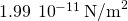What pressure does light emitted uniformly in all directions from a 100-W incandescent light bulb exert on a mirror at a distance of 3.0 m, if 2.6 W of the power is emitted as visible light?

A microscopic spherical dust particle of radius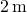and mass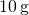is moving in outer space at a constant speed of 30 cm/sec. A wave of light strikes it from the opposite direction of its motion and gets absorbed. Assuming the particle decelerates uniformly to zero speed in one second, what is the average electric field amplitude in the light?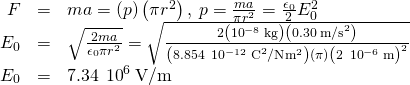A Styrofoam spherical ball of radius 2 mm and mass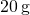is to be suspended by the radiation pressure in a vacuum tube in a lab. How much intensity will be required if the light is completely absorbed the ball?

Suppose that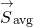for sunlight at a point on the surface of Earth is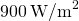. (a) If sunlight falls perpendicularly on a kite with a reflecting surface of area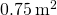, what is the average force on the kite due to radiation pressure? (b) How is your answer affected if the kite material is black and absorbs all sunlight?

a.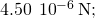b. it is reduced to half the pressure,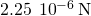Sunlight reaches the ground with an intensity of about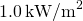. A sunbather has a body surface area of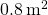facing the sun while reclining on a beach chair on a clear day. (a) how much energy from direct sunlight reaches the sunbather’s skin per second? (b) What pressure does the sunlight exert if it is absorbed?

Suppose a spherical particle of mass m and radius R in space absorbs light of intensity I for time t. (a) How much work does the radiation pressure do to accelerate the particle from rest in the given time it absorbs the light? (b) How much energy carried by the electromagnetic waves is absorbed by the particle over this time based on the radiant energy incident on the particle?

a.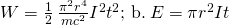### Glossary